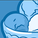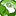# New to Qlik Sense

If you’re new to Qlik Sense, start with this Discussion Board and get up-to-speed quickly.

Announcements
cancel
Showing results for
Did you mean:Contributor II

## Calculation Sales growth

Hey all,

So I got a problem. I need to calculate sales number according to percentage growth of one year.

So for example my data contain that in 2022 my sales are 400 and the growth is 10 percent.

I want to calculate for each year how much the sales gonna be, so for 2023 440 and for 2024 484.and so on.

I can't right It on the script cause the numbers need change by the user.

Is there any way to calculate it?Creator III

Hi @Reuven12 ,

The calculation for compounding increases in Qlik uses the Pow( ) function.Projected 1 Year = sum(num(Amount * pow(1 + 0.1, 1)))

Projected 2 Year = sum(num(Amount * pow(1 + 0.1, 2)))

Projected 3 Year = sum(num(Amount * pow(1 + 0.1, 3)))

etc...

I'm not sure what you meant by the numbers changing per user, but this can be a start.

I hope it helps.

Thanks

AnthonyTags